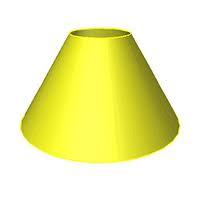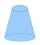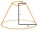# Cone

Circular cone with height h = 29 dm and base radius r = 3 dm slice plane parallel to the base. Calculate the distance of the cone vertex from this plane, if solids have the same volume.

x =  23 dm

### Step-by-step explanation:Did you find an error or inaccuracy? Feel free to write us. Thank you!Tips to related online calculators
Tip: Our volume units converter will help you with the conversion of volume units.

## Related math problems and questions:

• Rotary coneRotary cone whose height is equal to the circumference of the base, has a volume 229 cm3. Calculate the radius of the base circle and height of the cone.
• Right circular coneThe volume of a right circular cone is 5 liters. Calculate the volume of the two parts into which the cone is divided by a plane parallel to the base, one-third of the way down from the vertex to the base.
• 2x coneCircular cone height 84 cm was cut plane parallel with base. Volume of these two small cones is the same. Calculate the height of the smaller cone.
• CylinderThe cylinder surface is 922 dm2. Its height is equal to the radius of the base. Calculate the height of this cylinder.
• Cone and cubeAbout what percentage hase cone with base radius r larger volume than same high cube with square base with edge length r?
• Rotating coneCalculate the volume and the surface area of a rotating cone of base radius r = 2.3 dm and a height h = 46 mm.
• The volumeThe volume of the cone is 94.2dm³, the radius of the base is 6 dm Calculate the surface of the cone.
• Cube 9What was the cube's original edge length after cutting 39 small cubes with an edge of 2 dm left 200 dm3?
• The coneThe cone with a base radius of 12 cm and a height of 20 cm was truncated at a distance of 6 cm from the base, thus creating a truncated cone. Find the radius of the base of the truncated cone.
• ConeCircular cone of height 15 cm and volume 5699 cm3 is at one-third of the height (measured from the bottom) cut by a plane parallel to the base. Calculate the radius and circumference of the circular cut.
• Cone area and sideCalculate the surface area and volume of a rotating cone with a height of 1.25 dm and 17,8dm side.
• PrismRight-angled prism, whose base is a right triangle with leg a = 3 cm and hypotenuse c = 13 cm, has the same volume as a cube with an edge length of 3 dm. a) Find the height of the prism b) Calculate the surface of the prism c) What percentage of the cube'
• Triangular prismCalculate the volume and surface area of a triangular prism if it is given: a = 6.8 dm. ..Va = 4 dm. (base edge length and base triangle height length) ... ... .v = 23 dm (body height)
• Volume and surfaceCalculate the volume and surface area of the cylinder when the cylinder height and base diameter is in a ratio of 3:4 and the area of the cylinder jacket is 24 dm2.The regular quadrilateral pyramid has a base edge a = 1.56 dm and a height h = 2.05 dm. Calculate: a) the deviation angle of the sidewall plane from the base plane b) deviation angle of the side edge from the plane of the baseFind the surface of the cube with a volume of 27 dm3.A cone with a base radius of 10 cm and a height of 12 cm is given. At what height above the base should we divide it by a section parallel to the base so that the volumes of the two resulting bodies are the same? Express the result in cm.